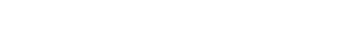# NCERT Solutions for Class 10 Maths Chapter 1 Real Numbers Ex 1.3

(Last Updated On: September 8, 2023)
NCERT Solutions for Class 10 Maths Chapter 1 Real Numbers Ex 1.3: Get free NCERT Solutions for Class 10 Maths Chapter 1 Pre 1.3 PDF. While doing homework , Real Numbers Class 10 Maths NCERT Solutions are extremely helpful . Exercise 1.3 Class 10 Maths NCERT Solutions were prepared by experienced NRACETJOB.com teachers. Detailed answers to all the questions in Chapter 1 Maths Class 10 Real Numbers Exercise 1.3 are given in the NCERT Text Book.

Topics and Sub Topics in Class 10 Maths Chapter 1 Real Numbers Ex 1.3:Section Name Topic Name 1 Real Numbers 1.1 Introduction 1.2 Euclid’s Division Lemma 1.3 The Fundamental Theorem of Arithmetic 1.4 Revisiting Irrational Numbers 1.5 Revisiting Rational Numbers and Their Decimal Expansions 1.6 Summary
• Class 10 Maths Real Numbers Ex 1.1
• प्रश्नावली 1.1 का हल हिंदी में वास्तविक संख्याएँ
• Class 10 Maths Real Numbers Ex 1.2
• प्रश्नावली 1.2 का हल हिंदी में वास्तविक संख्याएँ
• Class 10 Maths Real Numbers Ex 1.3
• प्रश्नावली 1.3 का हल हिंदी में वास्तविक संख्याएँ
• Class 10 Maths Real Numbers Ex 1.4
• प्रश्नावली 1.4  का हल हिंदी में वास्तविक संख्याएँ
• Real Numbers Class 10 Extra Questions

You can also download free PDF of Pre 1.3 Class 10 Actual Numbers NCERT Solutions or save solution images and take a print out to keep it handy for your exam preparation.

 Board CBSE Textbook NCERT Class Class 10 Subject Maths Chapter Chapter 1 Chapter Name Real Numbers Exercise Ex 1.3 Number of Questions Solved 3 Category NCERT Solutions

## NCERT Solutions for Class 10 Maths Chapter 1 Real Numbers Ex 1.3

NCERT Solutions for Class 10 Maths Chapter 1 Real Numbers Ex 1.3 are part of NCERT Solutions for Class 10 Maths. Here we have given the NCERT Book for Maths Class 10 Chapter 1 Real Numbers Exercise 1.3

Ex 1.3 Class 10 Maths Question 1.
Prove that √5 is irrational.
Solution:Ex 1.3 Class 10 Maths Question 2.
Show that 3 + √5 is irrational.
Solution:Ex 1.3 Class 10 Maths Question 3.
Prove that the following are irrational.Solution: